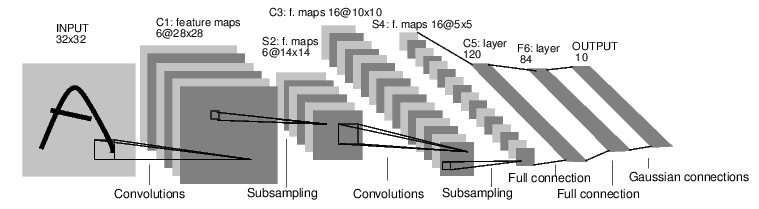# 神经网络

`autograd` 实现了反向传播功能, 但是直接用来写深度学习的代码在很多情况下还是稍显复杂, `torch.nn` 是专门为神经网络设计的模块化接口. `nn` 构建于 `Autograd` 之上, 可用来定义和运行神经网络. `nn.Module``nn` 中最重要的类, 可把它看成是一个网络的封装, 包含网络各层定义以及 `forward` 方法, 调用 `forward(input)` 方法, 可返回前向传播的结果.convnet

• 定义具有一些可学习参数(或权重)的神经网络
• 迭代输入数据集
• 通过网络处理输入
• 计算损失(输出的预测值与实际值之间的距离)
• 将梯度传播回网络
• 更新网络的权重, 通常使用一个简单的更新规则: `weight = weight - learning_rate * gradient`

## 定义网络

``````import torch
import torch.nn as nn
import torch.nn.functional as F

class Net(nn.Module):

def __init__(self):
super(Net, self).__init__()
# 卷积层 '1'表示输入图片为单通道, '6'表示输出通道数, '5'表示卷积核为5*5
# 核心
self.conv1 = nn.Conv2d(1, 6, 5)
self.conv2 = nn.Conv2d(6, 16, 5)
# 仿射层/全连接层: y = Wx + b
self.fc1 = nn.Linear(16 * 5 * 5, 120)
self.fc2 = nn.Linear(120, 84)
self.fc3 = nn.Linear(84, 10)

def forward(self, x):
#在由多个输入平面组成的输入信号上应用2D最大池化.
# (2, 2) 代表的是池化操作的步幅
x = F.max_pool2d(F.relu(self.conv1(x)), (2, 2))
# 如果大小是正方形, 则只能指定一个数字
x = F.max_pool2d(F.relu(self.conv2(x)), 2)
x = x.view(-1, self.num_flat_features(x))
x = F.relu(self.fc1(x))
x = F.relu(self.fc2(x))
x = self.fc3(x)
return x

def num_flat_features(self, x):
size = x.size()[1:]  # 除批量维度外的所有维度
num_features = 1
for s in size:
num_features *= s
return num_features

net = Net()
print(net)
``````

``````params = list(net.parameters())
print(len(params))
print(params.size())  # conv1的weight
``````

``````input = Variable(torch.randn(1, 1, 32, 32))
out = net(input)
print(out)
``````

``````net.zero_grad()
out.backward(torch.randn(1, 10))
``````

`torch.nn` 只支持小批量(mini-batches), 不支持一次输入一个样本, 即一次必须是一个 batch.

• `torch.Tensor` - 一个 多维数组.
• `autograd.Variable` - 包装张量并记录应用于其上的历史操作. 具有和 `Tensor` 相同的 API ,还有一些补充, 如 `backward()`. 另外 拥有张量的梯度.
• `nn.Module` - 神经网络模块. 方便的方式封装参数, 帮助将其移动到GPU, 导出, 加载等.
• `nn.Parameter` - 一种变量, 当被指定为 `Model` 的属性时, 它会自动注册为一个参数.
• `autograd.Function` - 实现 autograd 操作的向前和向后定义 . 每个 `Variable` 操作, 至少创建一个 `Function` 节点, 连接到创建 `Variable` 的函数, 并 编码它的历史.

• 定义一个神经网络
• 处理输入并反向传播

• 计算损失函数
• 更新网络的权重

## 损失函数

`nn` 包下有几种不同的 损失函数 . 一个简单的损失函数是: `nn.MSELoss` 计算输出和目标之间的均方误差

``````output = net(input)
target = Variable(torch.arange(1, 11))  # 一个虚拟的目标
criterion = nn.MSELoss()

loss = criterion(output, target)
print(loss)
``````

``````input -> conv2d -> relu -> maxpool2d -> conv2d -> relu -> maxpool2d
-> view -> linear -> relu -> linear -> relu -> linear
-> MSELoss
-> loss
``````

``````print(loss.grad_fn)  # MSELoss
``````

## 反向传播

``````net.zero_grad()     # 把之前的梯度清零

loss.backward()

``````

• 更新网络的权重

## 更新权重

`weight = weight - learning_rate * gradient`

``````learning_rate = 0.01
for f in net.parameters():
``````

``````import torch.optim as optim

# 新建一个优化器, 指定要调整的参数和学习率
optimizer = optim.SGD(net.parameters(), lr = 0.01)

# 在训练过程中: